# dsp.DigitalDownConverter

Translate digital signal from intermediate frequency (IF) band to baseband and decimate it

## Description

The `dsp.DigitalDownConverter` object translates digital signal from intermediate frequency (IF) band to baseband and decimates it.

To digitally downconvert the input signal:

1. Create the `dsp.DigitalDownConverter` object and set its properties.

2. Call the object with arguments, as if it were a function.

## Creation

### Syntax

``dwnConv = dsp.DigitalDownConverter``
``dwnConv = dsp.DigitalDownConverter(Name,Value)``

### Description

````dwnConv = dsp.DigitalDownConverter` returns a digital downconverter (DDC) System object™, `dwnConv`.```

example

``` `dwnConv = dsp.DigitalDownConverter(Name,Value)` returns a DDC object, `dwnConv`, with the specified property `Name` set to the specified `Value`. You can specify additional name-value pair arguments in any order as (`Name1`,`Value1`,...,`NameN`,`ValueN`). ```

## Properties

expand all

Unless otherwise indicated, properties are nontunable, which means you cannot change their values after calling the object. Objects lock when you call them, and the `release` function unlocks them.

If a property is tunable, you can change its value at any time.

Set this property to a positive scalar value, greater than or equal to twice the value of the `CenterFrequency` property.

Data Types: `single` | `double`

Set this property to a positive integer scalar, or to a 1-by-2 or 1-by-3 vector of positive integers.

When you set this property to a scalar, the object automatically chooses the decimation factors for each of the three filtering stages.

When you set this property to a 1-by-2 vector, the object bypasses the third filter stage and sets the decimation factor of the first and second filtering stages to the values in the first and second vector elements respectively. Both elements of the `DecimationFactor` vector must be greater than one.

When you set this property to a 1-by-3 vector, the i th element of the vector specifies the decimation factor for the ith filtering stage. The first and second elements of the `DecimationFactor` vector must be greater than one, and the third element must be 1 or 2.

Data Types: `double`

When you set this property to `true`, the object designs filters with the minimum order that meets the passband ripple, stopband attenuation, passband frequency, and stopband frequency specifications that you set using the `PassbandRipple`, `StopbandAttenuation`, `Bandwidth`, `StopbandFrequencySource`, and `StopbandFrequency` properties.

When you set this property to `false`, the object designs filters with orders that you specify in the `NumCICSections`, `SecondFilterOrder`, and `ThirdFilterOrder` properties. The filter designs meet the passband and stopband frequency specifications that you set using the `Bandwidth`, `StopbandFrequencySource`, and `StopbandFrequency` properties.

Data Types: `logical`

Number of sections of CIC decimator, specified as a positive integer scalar.

#### Dependencies

This property applies when you set the `MinimumOrderDesign` property to `false`.

Data Types: `double`

Order of CIC compensation filter stage, specified as a positive integer scalar.

#### Dependencies

This property applies when you set the `MinimumOrderDesign` property to `false`.

Data Types: `double`

Order of third filter stage, specified as an even positive integer scalar. When you set the `DecimationFactor` property to a 1-by-2 vector, the object ignores the `ThirdFilterOrder` property because the third filter stage is bypassed.

#### Dependencies

This property applies when you set the `MinimumOrderDesign` property to `false`.

Data Types: `double`

Two-sided bandwidth of input signal in Hz, specified as a positive integer scalar. The object sets the passband frequency of the cascade of filters to one-half of the value that you specify in the `Bandwidth` property. Set the value of this property to less than `SampleRate`/`DecimationFactor`.

Data Types: `double`

Specify the source of the stopband frequency as one of `Auto` | `Property`. When you set this property to `Auto`, the object places the cutoff frequency of the cascade filter response at approximately``` Fc = SampleRate/M/2``` Hz, where M is the total decimation factor that you specify in the `DecimationFactor` property. The object computes the stopband frequency as ```Fstop = Fc + TW/2```. TW is the transition bandwidth of the cascade response computed as `2×(Fc–Fp)`, and the passband frequency, Fp, equals `Bandwidth/2`.

Stopband frequency in Hz, specified as a positive scalar.

#### Dependencies

This property applies when you set the `StopbandFrequencySource` property to `Property`.

Data Types: `double`

Passband ripple of cascade response in dB, specified as a positive scalar. When you set the `MinimumOrderDesign` property to `true`, the object designs the filters so that the cascade response meets the passband ripple that you specify in the `PassbandRipple` property.

#### Dependencies

This property applies when you set the `MinimumOrderDesign` property to `true`.

Data Types: `double`

Stopband attenuation of cascade response in dB, specified as a positive scalar. When you set the `MinimumOrderDesign` property to `true`, the object designs the filters so that the cascade response meets the stopband attenuation that you specify in the `StopbandAttenuation` property.

#### Dependencies

This property applies when you set the `MinimumOrderDesign` property to `true`.

Data Types: `double`

Specify the oscillator as one of `Sine wave` | `NCO` | `Input port` | `None`. When you set this property to `Sine wave`, the object frequency down converts the input signal using a complex exponential obtained from samples of a sinusoidal trigonometric function. When you set this property to `NCO`, the object performs frequency down conversion with a complex exponential obtained using a numerically controlled oscillator (NCO). When you set this property to ```Input port```, the object performs frequency down conversion using the complex oscillator signal, `z`, that you pass as an input to the object. When you set this property to `None`, the mixer stage in the object is not present and the object acts as three stage cascaded decimator.

Center frequency of input signal in Hz, specified as a positive scalar that is less than or equal to half the value of the `SampleRate` property. The object down converts the input signal from the passband center frequency you specify in the `CenterFrequency` property, to `0` Hz.

#### Dependencies

This property applies when you set the `Oscillator` property to `Sine wave` or `NCO`.

Data Types: `double`

Number of NCO accumulator bits, specified as a positive integer in the range `[1 128]`.

#### Dependencies

This property applies when you set the `Oscillator` property to `NCO`.

Data Types: `double`

Number of NCO quantized accumulator bits, specified as an integer scalar in the range `[1 128]`. The value you specify in this property must be less than the value you specify in the `NumAccumulatorBits` property.

#### Dependencies

This property applies when you set the `Oscillator` property to `NCO`.

Data Types: `double`

When you set this property to `true`, a number of dither bits specified in the `NumDitherBits` property will be used to apply dither to the NCO signal.

#### Dependencies

This property applies when you set the `Oscillator` property to `NCO`.

Specify this property as an integer scalar smaller than the number of accumulator bits that you specify in the `NumAccumulatorBits` property.

#### Dependencies

This property applies when you set the `Oscillator` property to `NCO` and the Dither property to `true`.

Data Types: `double`

### Fixed-Point Properties

Specify the data type at the input of the first, second, and third (if it has not been bypassed) filter stages as one of `Same as input` | `Custom`. The object casts the data at the input of each filter stage according to the value you set in this property.

Specify the filters input fixed-point type as a scaled `numerictype` (Fixed-Point Designer) object with a Signedness of `Auto`.

#### Dependencies

This property applies when you set the `FiltersInputDataType` property to `Custom`.

Specify the data type of output as `Same as input` | `Custom`.

Specify the output fixed-point type as a scaled `numerictype` (Fixed-Point Designer) object with a Signedness of `Auto`.

#### Dependencies

This property applies when you set the `OutputDataType` property to `Custom`.

## Usage

### Syntax

``y = dwnConv(x)``
``y = dwnConv(x,z)``

### Description

example

``` `y = dwnConv(x)` takes an input `x` and outputs a signal, `y` that is frequency downconverted and downsampled.```
````y = dwnConv(x,z)` uses the complex input, `z`, as the oscillator signal used to frequency down convert input `x` when you set the `Oscillator` property to `Input port`.```

### Input Arguments

expand all

Data input, specified as a column vector or a matrix. The length of input `x` must be a multiple of the decimation factor. When the data type of `x` is `double` or `single` precision, the data type of `y` is the same as that of `x`. When the data type of `x` is of a fixed-point type, the data type of `y` is defined by the `OutputDataType` property.

The input can have multiple channels only if its data type is `double` or `single`. The input can be of data type `double`, `single`, signed integer, or signed fixed-point (`fi` objects).

Data Types: `single` | `double` | `int8` | `int16` | `int32` | `int64` | `fi`
Complex Number Support: Yes

Oscillator signal used to frequency down convert the input signal, specified as a column vector or a matrix. This input must be complex. The length of `z` must be equal to the length of `x`. `z` can be `double`, `single`, signed integer, or signed fixed-point (`fi` objects).

#### Dependencies

This input applies when you set the `Oscillator` property to `Input port`.

Data Types: `single` | `double` | `int8` | `int16` | `int32` | `int64` | `fi`
Complex Number Support: Yes

### Output Arguments

expand all

Down converted and down sampled signal, returned as a column vector or a matrix. The length of `y` is equal to the length of `x` divided by the `DecimationFactor`. When the data type of `x` is `double` or `single` precision, the data type of `y` is the same as that of `x`. When the data type of `x` is of a fixed point type, the data type of `y` is defined by the `OutputDataType` property.

Data Types: `single` | `double` | `int8` | `int16` | `int32` | `int64` | `fi`
Complex Number Support: Yes

## Object Functions

To use an object function, specify the System object as the first input argument. For example, to release system resources of a System object named `obj`, use this syntax:

`release(obj)`

expand all

 `getDecimationFactors` Get decimation factors of each filter stage of a digital down converter `getFilterOrders` Get orders of digital down converter or digital up converter filter cascade `getFilters` Get handles to digital down converter or digital up converter filter cascade objects `fvtool` Visualize frequency response of digital down converter or digital up converter filter cascade `groupDelay` Group delay of digital down converter or digital up converter filter cascade `visualizeFilterStages` Display response of digital down converter or digital up converter filter cascade `generatehdl` Generate HDL code for quantized DSP filter (requires Filter Design HDL Coder)
 `step` Run System object algorithm `release` Release resources and allow changes to System object property values and input characteristics `reset` Reset internal states of System object

## Examples

collapse all

Create a digital up converter object that up samples a 1 KHz sinusoidal signal by a factor of 20 and up converts it to 50 KHz. Create a digital down converter object that down converts the signal to 0 Hz and down samples it by a factor of 20.

Note: If you are using R2016a or an earlier release, replace each call to the object with the equivalent step syntax. For example, `obj(x)` becomes `step(obj,x)`.

Create a sine wave generator to obtain the 1 KHz sinusoidal signal with a sample rate of 6 KHz.

```Fs = 6e3; % Sample rate sine = dsp.SineWave('Frequency',1000,'SampleRate',... Fs,'SamplesPerFrame',1024); x = sine(); % generate signal```

Create a `DigitalUpConverter` object. Use minimum order filter designs and set passband ripple to 0.2 dB and the stopband attenuation to 55 dB. Set the double sided signal bandwidth to 2 KHz.

```upConv = dsp.DigitalUpConverter(... 'InterpolationFactor', 20,... 'SampleRate', Fs,... 'Bandwidth', 2e3,... 'StopbandAttenuation', 55,... 'PassbandRipple',0.2,... 'CenterFrequency',50e3);```

Create a `DigitalDownConverter` object. Use minimum order filter designs and set the passband ripple to 0.2 dB and the stopband attenuation to 55 dB.

```dwnConv = dsp.DigitalDownConverter(... 'DecimationFactor',20,... 'SampleRate', Fs*20,... 'Bandwidth', 3e3,... 'StopbandAttenuation', 55,... 'PassbandRipple',0.2,... 'CenterFrequency',50e3);```

Create a spectrum estimator to visualize the signal spectrum before up converting, after up converting, and after down converting.

```window = hamming(floor(length(x)/10)); figure; pwelch(x,window,[],[],Fs,'centered') title('Spectrum of baseband signal x')```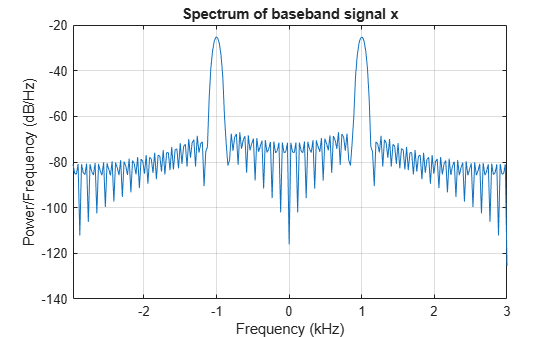Up convert the signal and visualize the spectrum

```xUp = upConv(x); % up convert window = hamming(floor(length(xUp)/10)); figure; pwelch(xUp,window,[],[],20*Fs,'centered'); title('Spectrum of up converted signal xUp')```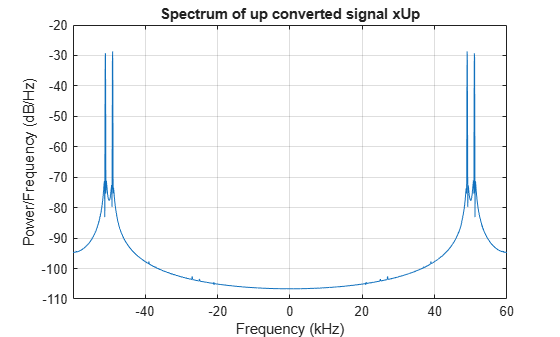Down convert the signal and visualize the spectrum

```xDown = dwnConv(xUp); % down convert window = hamming(floor(length(xDown)/10)); figure; pwelch(xDown,window,[],[],Fs,'centered') title('Spectrum of down converted signal xDown')```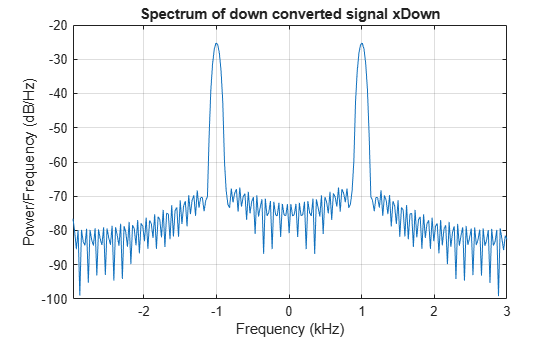Visualize the response of the decimation filters

`visualizeFilterStages(dwnConv)`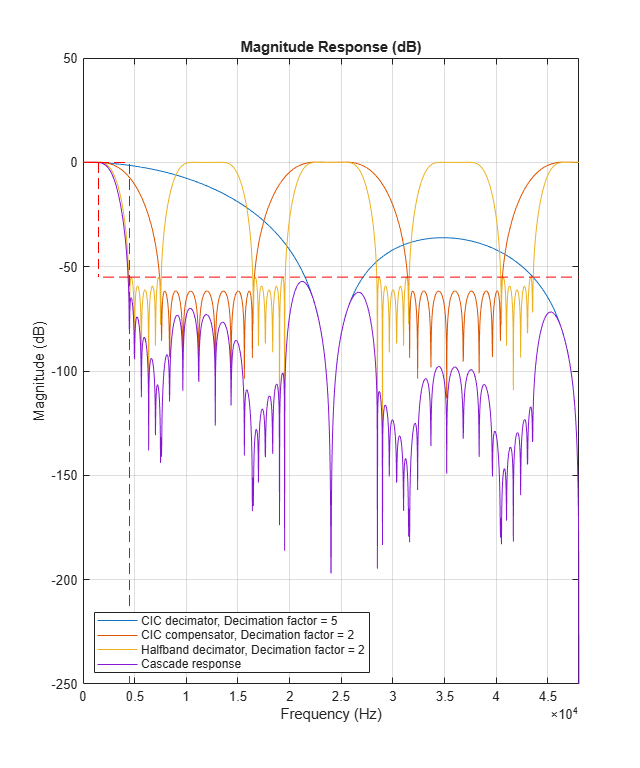Get decimation factors of each filter stage of the `dsp.DigitalDownConverter` System object™.

Create a `dsp.DigitalDownConverter` System object with the default settings. Using the `getDecimationFactors` function, obtain the decimation factors of each stage of the object.

`dwnConv = dsp.DigitalDownConverter`
```dwnConv = dsp.DigitalDownConverter with properties: DecimationFactor: 100 MinimumOrderDesign: true Bandwidth: 200000 StopbandFrequencySource: 'Auto' PassbandRipple: 0.1000 StopbandAttenuation: 60 Oscillator: 'Sine wave' CenterFrequency: 14000000 SampleRate: 30000000 Show all properties ```
`M = getDecimationFactors(dwnConv) %#ok`
```M = 1×3 25 2 2 ```

The `DecimationFactor` property of the object is set to 100. The output `M` is by default a 1-by-3 vector, where each element in the vector is a factor of the overall decimation factor.

When you set the `DecimationFactor` to a 1-by-2 vector, the object bypasses the third filter stage and sets the decimation factor of the first and second filtering stages to the values in the first and second vector elements respectively.

`dwnConv.DecimationFactor = [10 10]`
```dwnConv = dsp.DigitalDownConverter with properties: DecimationFactor: [10 10] MinimumOrderDesign: true Bandwidth: 200000 StopbandFrequencySource: 'Auto' PassbandRipple: 0.1000 StopbandAttenuation: 60 Oscillator: 'Sine wave' CenterFrequency: 14000000 SampleRate: 30000000 Show all properties ```
`M = getDecimationFactors(dwnConv)`
```M = 1×2 10 10 ```

The output of the `getDecimationFactors` function is now a 1-by-2 vector.

## Algorithms

The object downconverts the input signal by multiplying it with a complex exponential with center frequency equal to the value in the `CenterFrequency` property. The object downsamples the frequency downconverted signal using a cascade of three decimation filters. In this case, the filter cascade consists of a CIC decimator, a CIC compensator, and a third FIR decimation stage. The following block diagram shows the architecture of the digital down converter.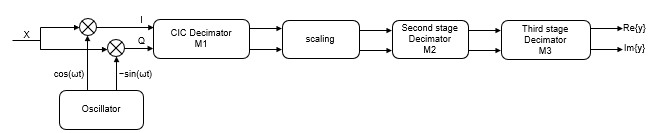The scaling section normalizes the CIC gain and the oscillator power. It may also contain a correction factor to achieve the desired ripple specification. When you set the Oscillator property to `InputPort`, the normalization factor does not include the oscillator power factor. Depending on the setting of the DecimationFactor property, you may be able to bypass the third filter stage. When the input data type is double or single, the object implements an N-section CIC decimation filter as an FIR filter with a response that corresponds to a cascade of N boxcar filters. A true CIC filter with actual comb and integrator sections is implemented when the input data is of a fixed-point type. The CIC filter is emulated with an FIR filter so that you can run simulations with floating-point data.

The following block diagram represents the DDC arithmetic with single or double-precision, floating-point inputs.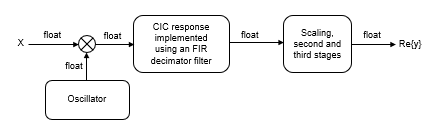For details of fixed-point operation, see Fixed Point.

## Extended Capabilities

Introduced in R2012a

## SupportGet trial now